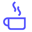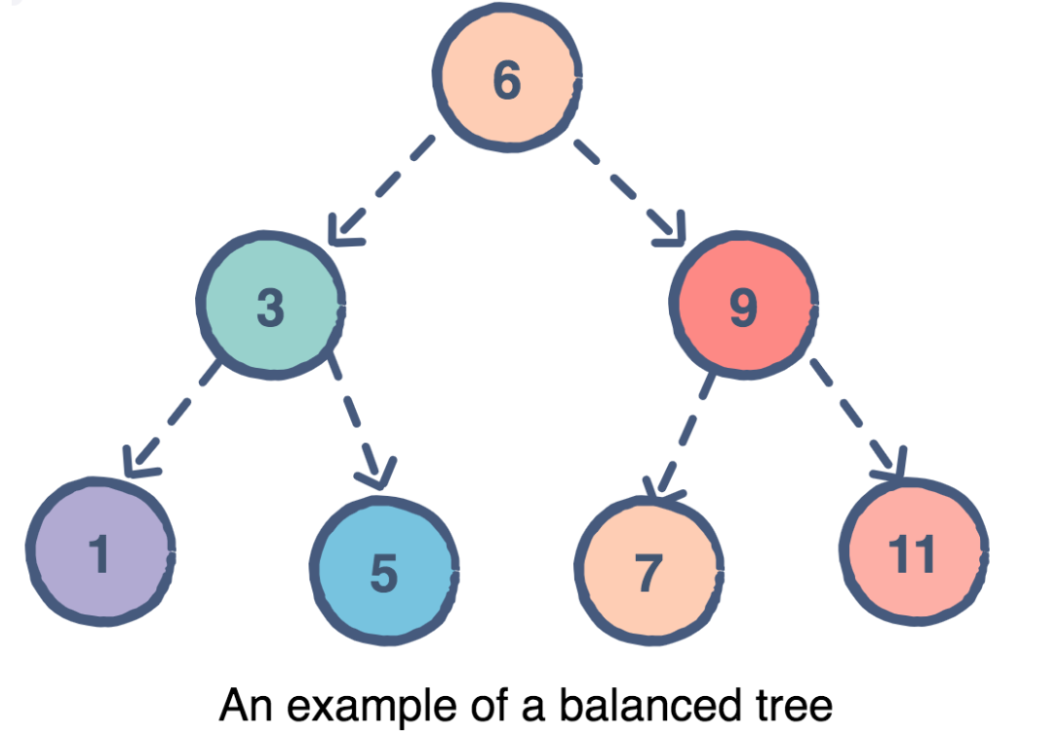a concise shot of dev knowledge
Become a Contributor
Concise shots of dev knowledge

RELATED TAGS

avl
data structures

# What is an AVL tree?Edpresso Team

AVL, named after inventors Adelson-Velsky and Landis, is a binary tree that​ self-balances by keeping a check on the balance factor of every node.

## Balance Factor

The balance factor of a node is the difference in the heights of the left and right subtrees. The balance factor of every node in the AVL tree should be either +1, 0 or -1.After each insertion or deletion, if the balance factor of any node does not follow the AVL balance property, the AVL tree balances itself through the following rotation techniques:

• Left Rotation
• Right Rotation
• Left-Right Rotation
• Right-Left Rotation

## Time Complexity

Due to the balancing property, the insertion, deletion and search operations take $O(log n)$ in both the average and the worst cases. Therefore, AVL trees give us an edge over Binary Search Trees which have an $O(n)$ time complexity in the worst case scenario.

## Space Complexity

The space complexity of an AVL tree is $O(n)$ in both the average and the worst case.

RELATED TAGS

avl
data structures
RELATED COURSES

View all Courses

Keep Exploring

Learn in-demand tech skills in half the time